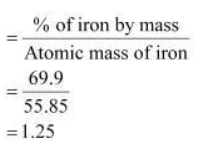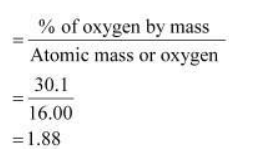# Determine the empirical formula of an oxide of iron which has 69.9%

Question.

Determine the empirical formula of an oxide of iron which has 69.9% iron and 30.1% dioxygen by mass

Solution:

% of iron by mass = 69.9 % [Given]

% of oxygen by mass = 30.1 % [Given] Relative

moles of iron in iron oxideRelative moles of oxygen in iron oxideSimplest molar ratio of iron to oxygen

= 1.25: 1.88

= 1: 1.5

= 2: 3

$\therefore$ The empirical formula of the iron oxide is $\mathrm{Fe}_{2} \mathrm{O}_{3}$.